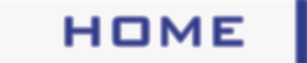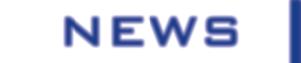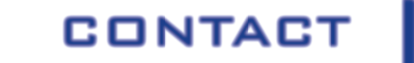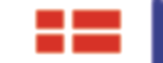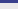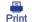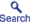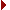Measure the thickness of a hair Demonstration no.: 87 Purpose: To measure the thickness of a piece of hair using a laser beam. Summary: A laser beam (from for instance a laser pointer) is directed towards a piece of hair. This creates a diffraction pattern, from which the diameter of the hair can be measured. Keywords: Electromagnetic radiation, refraction, diffraction, interference, light, optics. Description: The diameter of a hair (or a thin string or electrical wire) can be measured by scattering laser light on the hair. The scattering creates a diffraction pattern consisting of a line of light and dark spots (see the picture further down below).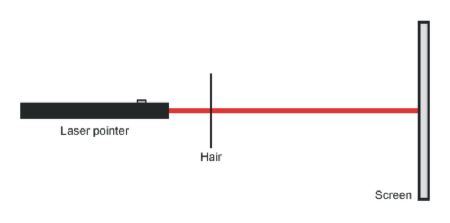Laser light is scattered on a hair, thereby creating a diffraction pattern which can be used to determine the thickness of the hair. If you simply measure the distance from the hair to the projection screen, and the distance from the centre to one of the dark spots, the diameter of the hair can be calculated.A piece of hair is attached to a laser pointer using tape. According to "Babinet's Principle", the diffraction pattern of a narrow aperture is the same as that of a narrow screen of the same width as the aperture. So when one needs to calculate the diffraction pattern of a hair, the same equations as those for a narrow aperture can be used.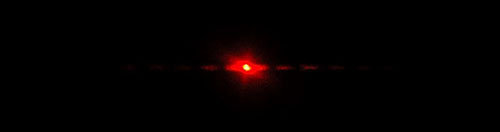Diffraction pattern from red laser light scattered from a hair. By measuring the distance from the center to one of the dark bands, the thickness of the hair can be determined. If the first dark band is considered, you can start by calculating the angle to this as seen from the hair. Measure the distance from the hair to the projection screen (l) and from the centre of the diffraction pattern to the first dark band (x). The angle can be calculated from this equation: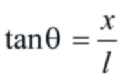Equation used to find the angle to the dark bands of the diffraction pattern. When the angle theta is known, the diameter of the hair (w) can be found from the following equation using the wavelength lambda of the laser: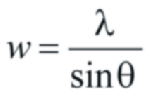Equation used to find the diameter of a hair. The experiment is well-suited as a student exercise, where the students determine hair thicknesses of everyone in a class. How does the thickness vary with sex, race, ages and hair color? For that purpose, one can download the program "laserWidth", which can be found in the references. To test if the method works, one can start by measuring the thickness of a thin metal wire of known diameter (e.g. a 0.1 mm metal wire). In this way, the students can test if they understand how to perform the measurement correctly. The demonstration should also be performed on both straight and curly hair. Several measurements should be made, where the angle of scattered laser light is varied. This will show that curly hair has a more oval cross section, while straight hair is more round! As described in the references, one can also use laser light diffraction to measure sizes of other object like for instance blood cells or pollen. Equipment and materials:LaserRuler / tape measure References:C. Bowlt: "Measurement of red blood cell diameters using a laser", Phys. Educ. 6, 13 (1971). (http://www.iop.org/EJ/journal/0031-9120)S.M. Curry and A.L. Shawlow: "Measuring the diameter of a hair by diffraction", Am. J. Phys. 42, 412 (1974).Y.P. Hwu: "Diffraction pattern of a hair", Am. J. Phys. 45, 404 (1977).T.B. Greenslade, Jr.: "Diffraction by a cat's whisker", The Physics Teacher 38, 422 (2000). (http://scitation.aip.org/tpt)Link to demonstration in database at University of Michigan.Link to demonstration in database at University of Iowa.Download "laserWidth" (Software to calculate the diameter of a hair). PIRA DCS: 6C20.20 (Optics: Diffraction)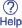Updated: 27 October 2007 If you have comments, corrections or suggestions regarding this demonstration, please us at FYSIKBASEN.DK.